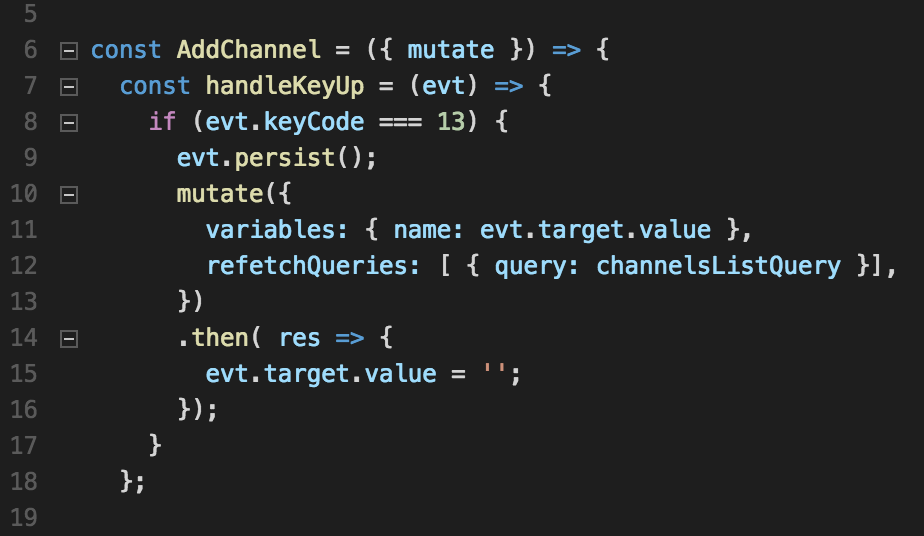## Tutorial: 在React中使用GraphQL mutation1.将我们的前端连接到服务器 2.在服务器上定义GraphQL变化 3.从客户端上的React组件调用GraphQL变体

``````git clone https://github.com/apollographql/graphql-tutorial.gitcd graphql-tutorialgit fetchgit checkout t3-start
``````

``````cd servernpm install && npm start
``````
``````# ...# GraphQL Server is now running on http://localhost:4000/graphiql
``````

``````# assuming that you're in the graphql-tutorial directory
``````
``````cd clientnpm install && npm start
``````
``````# ...# The app is running at:##    http://localhost:3000/
``````

#### 1. 将前端连接到服务器

``````# in the server directory (not the client!!)
``````
``````npm install --save cors
``````

``````// ...import cors from 'cors';
``````
``````// ... const server = express();server.use('*', cors({ origin: 'http://localhost:3000' }));
``````

``````// client/src/App.js
``````
``````import {  ApolloClient,  ApolloProvider,  createNetworkInterface, // <-- this line is new!} from 'react-apollo';
``````

``````const networkInterface = createNetworkInterface({   uri: 'http://localhost:4000/graphql',});
``````
``````const client = new ApolloClient({  networkInterface,});
``````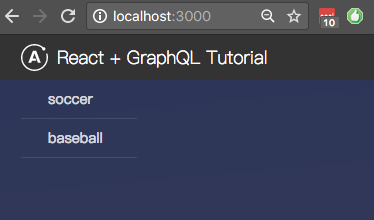#### 2. 在服务器上定义GraphQL mutation

``````const typeDefs = type Channel {  id: ID!                # "!" denotes a required field  name: String}type Query {  channels: [Channel]    # "[]" means this is a list of channels}
``````
``````# The mutation root type, used to define all mutations.type Mutation {  # A mutation to add a new channel to the list of channels  addChannel(name: String!): Channel};
``````

``````# an example mutation call:mutation {  addChannel(name: "basketball"){    id    name  }}
``````

``````const channels = /* ... */let nextId = 3;
``````
``````export const resolvers = {  Query: {    channels: () => {      return channels;
},  },  Mutation: {    addChannel: (root, args) => {      const newChannel = { id: nextId++, name: args.name };
channels.push(newChannel);
return newChannel;
},  },};
``````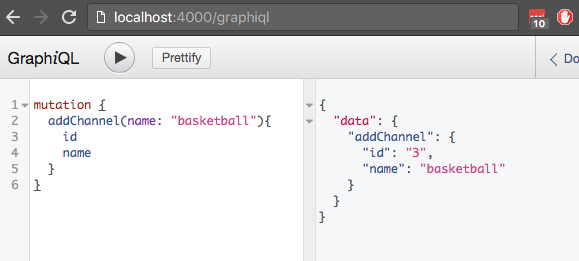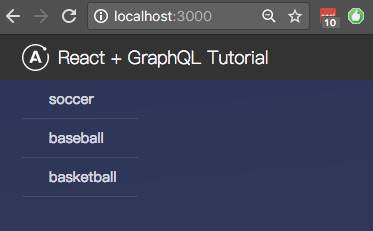Note: channels目前仅存储在内存中，因此每次重新启动服务器时，新添加的channel都将消失。 我们将在未来的教程中将事物与持久存储挂钩。

#### 3. 从React组件调用 mutation

``````import React from 'react';
``````
``````const AddChannel = () => {  const handleKeyUp = (evt) => {    if (evt.keyCode === 13) {      console.log(evt.target.value);
evt.target.value = '';
}  };
``````
``````  return (      );};
``````
``````export default AddChannel;
``````

``````import AddChannel from './AddChannel';
``````
``````// ...
``````
``````const ChannelsList = ({ data: {loading, error, channels }}) => {  if (loading) {    return Loading ...;
}  if (error) {    return {error.message};
}
``````
``````  return (           // <-- This is the new line.      { channels.map( ch =>         ({ch.name})      )}      );};
``````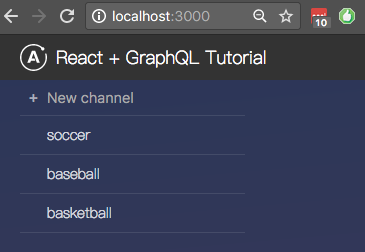``````import React from 'react';import { gql, graphql } from 'react-apollo';
``````
``````const AddChannel = ({ mutate }) => {  const handleKeyUp = (evt) => {    if (evt.keyCode === 13) {      evt.persist();
mutate({         variables: { name: evt.target.value }      })      .then( res => {        evt.target.value = '';
});
}  };
``````
``````return (      );};
``````
``````const addChannelMutation = gql  mutation addChannel(\$name: String!) {    addChannel(name: \$name) {      id      name    }  };
``````
``````const AddChannelWithMutation = graphql(  addChannelMutation)(AddChannel);
``````
``````export default AddChannelWithMutation;
``````

#### 4. 更新客户端状态使用mutation

1. Refetch 可能受mutation影响的查询
2. 基于mutation结果手动 更新客户端状态
3. 使用 GraphQL 订阅 来通知我们更新

``````// AddChannel.js
``````
``````// ...import { channelsListQuery } from './ChannelsListWithData';
``````
``````// ...
``````
``````    mutate({       variables: { name: evt.target.value },      refetchQueries: [ { query: channelsListQuery }], // <-- new    })
``````
``````// ...
``````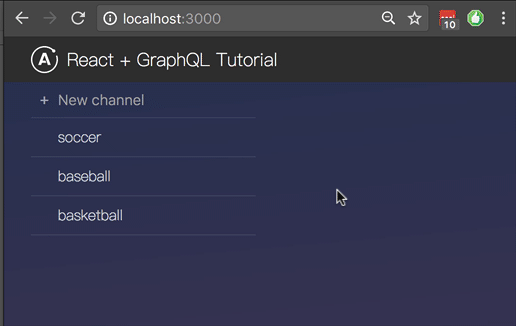``````export default graphql(channelsListQuery, {  options: { pollInterval: 5000 },})(ChannelsList);
``````